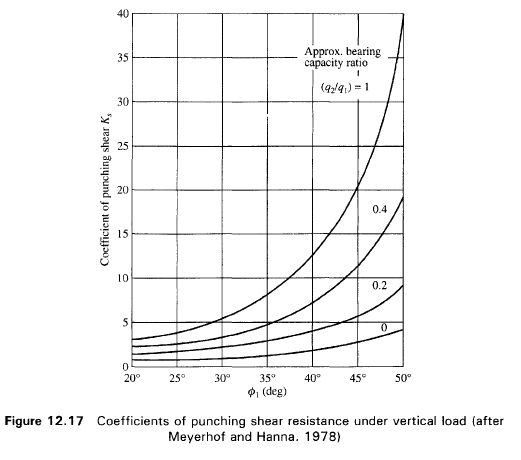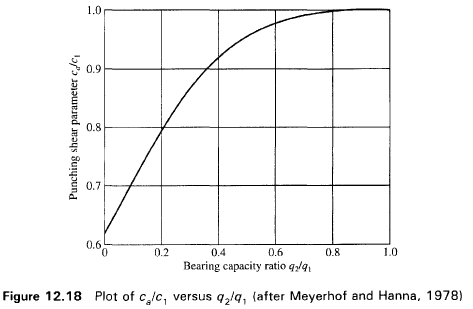Meyerhof Hanna 1978: Ultimate Bearing Capacity of Footings Resting on Stratified Deposits of Soil: All the theoretical analysis dealt with so far is based on the assumption that the subsoil is isotropic and homogeneous to a considerable depth. In nature, soil is generally non-homogeneous with mixtures of sand, silt and clay in different proportions. In the analysis, an average profile of such soils is normally considered. However, if soils are found in distinct layers of different compositions and strength characteristics, the assumption of homogeneity to such soils is not strictly valid if the failure surface cuts across boundaries of such layers.

The present analysis is limited to a system of two distinct soil layers. For a footing located in the upper layer at a depth D, below the ground level, the failure surfaces at ultimate load may either lie completely in the upper layer or may cross the boundary of the two layers. Further, we may come across the upper layer strong and the lower layer weak or vice versa . In either case, a general analysis for (c = 0) will be presented and will show the same analysis holds true if the soil layers are any one of the categories belonging to sand or clay.

The bearing capacity of a layered system was first analyzed by Button (1953) who considered only saturated clay (φ = 0). Later on Brown and Meyerhof (1969) showed that the analysis of Button leads to unsafe results. Vesic (1975) analyzed the test results of Brown and Meyerhof and others and gave his own solution to the problem.

Vesic considered both the types of soil in each layer, that is clay and (c = 0) soils. However, confirmations of the validity of the analysis of Vesic and others are not available. Meyerhof (1974) analyzed the two layer system consisting of dense sand on soft clay and loose sand on stiff clay and supported his analysis with some model tests.
Again Meyerhof and Hanna (1978) advanced the earlier analysis of Meyerhof (1974) to encompass (c = 0) soil and supported their analysis with model tests. The present section deals briefly with the analyses of Meyerhof (1974) and Meyerhof and Hanna (1978).

Case 1: A Stronger Layer Overlying a Weaker Deposit

Figure 12.16(a) shows a strip footing of width B resting at a depth D, below ground surface in a strong soil layer (Layer 1). The depth to the boundary of the weak layer (Layer 2) below the base of the footing is H. If this depth H is insufficient to form a full failure plastic zone in Layer 1 under the ultimate load conditions, a part of this ultimate load will be transferred to the boundary level mn. This load will induce a failure condition in the weaker layer (Layer 2). However, if the depth H is relatively large then the failure surface will be completely located in Layer 1 as shown in Fig. 12.16b.
The ultimate bearing capacities of strip footings on the surfaces of homogeneous thick beds of Layer 1 and Layer 2 may be expressed as:

Layer 1
q1=c1Nc1+0.5γ1BNγ1

Layer 2
q2=c2Nc2+0.5γ2BNγ2
Where:
Nc1, Nγ1= bearing capacity factors for soil in layer 1 for friction angle φ1
Nc2, Nγ2= bearing capacity factors for soil in layer 2 for friction angle φ2

for thr footing founded at a depth Df if the complete failure surface lies within the upper stronger Layer 1 (Fig 12.16(b)) an expression for ultimate bearing capacity of the upper layer may be wrtìitten as

qu=qt=c1Nc1+q’0Nγ10.5γ1BNγ1

If q1 is much greater that q2 and if the depth H is insufficient to form a full failure plastic condition in Layer 1, then the failure of the footing may be considered due to pushing of soil within the boundary ad and be through the top layer into the weak layer. The resisting force for punching may be assumed to develop on the faces ad and be passing through the edges of the footing. The forces that act on these surfaces are (per unit length of footing),qu=qb+2(Ca+Ppsinδ)/B-γ1H

Where:
qb= ultimate bearing capacity of layer 2
Pp= passive earth pressure
δ= inclination of Pp with the normal
Pp=[γ1H2/(2cosδ)](1+2Df/H)Kpsubstituting for Pp and Ca the equation for qu may be written as

qu=qb+2CaH/B+γ1H2/B[1+(2Df/H)]Kptanδ-γ1H

In  practice, it is convenient to use a coefficient Ks of punching shearing resistance on the vertical plane through the footing edges so that

Kstanφ1=Kptanδ

qu may be written as
qu=qb+2CaH/B+γ1H2/B[1+(2Df/H)]Kstanφ11H ≤ qt

Figure 12.17 gives the value of Ks for various values of φ1 as a  functionn of q2/q1. The variation of ca/c1 with q2/q1 is shown in Fig 12.18.

Introducing the hape factor for the corresponding layers with subscripts 1 and 2 :

qt=c1Nc1sc11DfNq1sq1+0.5γ1BNγ1sγ1

qb=c2Nc2sc21(Df+H)Nq2sq2+0.5γ2BNγ2sγ2

Case 2: Top layer Dense sand and Botton layer Saturated Soft Clay (φ2=0)

The value of qb for the bottom layer may be expressed as:

qb=c2Nc2sc21(Df+H)

for φ=0

qb=1+0.2(B/L)5.14c21(Df+H)

for c1=0

qt1DfNq1sq1+0.5γ1BNγ1sγ1

We may now an expression for qu as

qu=1+0.2(B/L)5.14c21H2/B[1+(2Df/H)][1+B/L]Kstanφ11Df ≤ γ1DfNq1sq1+0.5γ1BNγ1sγ1

The ratio of q2/q1may expressed by

q2/q1=5.14c2/(0.5γ1BNγ1)

Case 3: When layer 1 is dense Sand and layer 2 is Loose Sand (c1=c2=0)

The expression for qu for a rectangular footing may be expressed

qu1(Df+H)Nq2sq2+0.5γ2BNγ2sγ21H2/B[1+(2Df/H)][1+B/L]Kstanφ11H ≤ qt

where qt1DfNq1sq1+0.5γ1BNγ1sγ1

q2/q12Nγ21Nγ1

Case 4: Layer 1 is Stiff Saturated Clay (φ1=0) and layer 2 is Saturated Soft Clay (φ2=0)

The ultimate bearing capacity of the layered system can be gives as

qu=1+0.2(B/L)5.14c2+(1+B/L)(2CaH/B)+γ1Df ≤ qt

qt=1+0.2(B/L)5.14c1γ1Df

where
q2/q1=c2/c1

BEARING CAPACTY AND SETTLEMENTS LOADCAP SOFTWARE